0

# Numerical accuracy question

Hi everyone,

I would like to attract your attention to the problem that i have encountered as described below:

I have been working to convert input from Wema type fuel level sensor (resistance output) converting it into VIN for MD3 input using volt divider. After calibrating actual device I have defined 2 square curves (0-50% and 50%-100%) to get better accuracy. IQAN program attached (.FuelLevel.idax). When I opened this program in IQANDesign 5.08 I have got the following result when simulating on computer which you can see it is wrong result as and simulation would give only -039 or 1.04.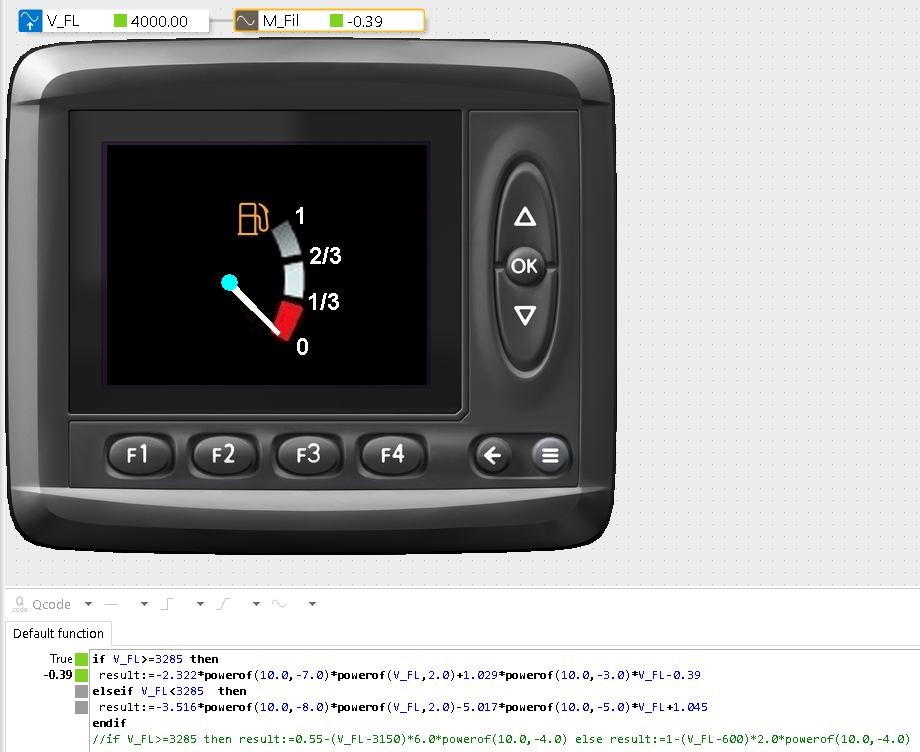If I load program in this stage into machine it would behave incorrect as in simulation

Then after playing with it I have discovered that closing simulation and then editing 'Default function' for M_Fil (Math channel) for example changing "-0.39" into "-0.391" and then back into "-0.39" and then restarting simulation it would come back to normal (what i would expect) as you can see on the following picture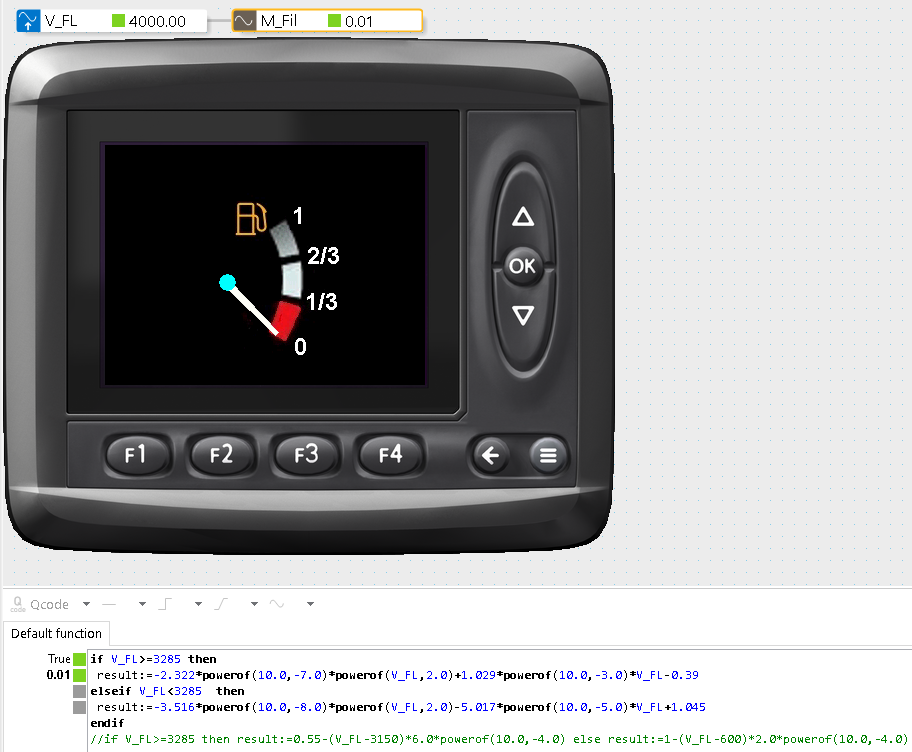When I have loaded the program into machine in this stage it has been working ok since then.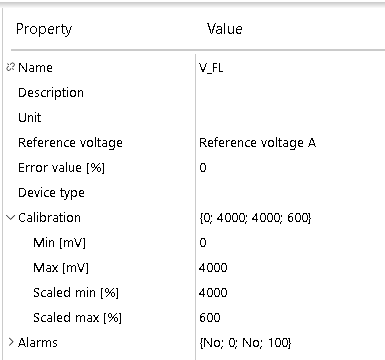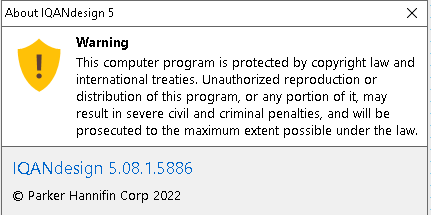Kind regards,
Richard Kowalczyk MIEAust CPEng(Ret)
32 Kingussie Ave
Castle Hill NSW 2154
Australia
📲 :+61 (0)431 639 295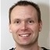+1

I took the liberty of changing the title from "MD3 with IQANDesign 5.08" to "numerical accuracy question", and changed topic type from bug to question.

The problem you get with this application is when you use the PowerOf() function to calculate very small numbers, you may end up with the value zero. The calculations are done with 32-bit real numbers.

A better solution is to make the calculation of your constants offline, for example like this:

if V_FL>=3285 then
result:=-0.0000002322 * PowerOf(V_FL,2) +0.001029 * V_FL -0.39
elseif V_FL<3285 then
result:=-0.00000003516 * PowerOf(V_FL,2) -0.00005017 * V_FL +1.045
endif

Another solution if it is still too sensitive to numerical accuracy is to implement using two lookup tables instead.+1

Thanks Gustav,

Your suggested solution works every time. Iwas puzzled that it gave me wrong results only when opening first time in IQANDesign and then in simulation. However, it worked always ok in simulation after performing editing function as described above.

Thanks for corrections

Take care

Richard